GFG App
Open AppBrowser
Continue

Excel is among the most powerful and widely used spreadsheet tools which eases organizing numbers and data within the sheets with pre-built formulas and functions. In this article, we are going to study Array Formulas. Arrays are one of them. Using Excel Array Formulas, make it super easy to handle a lot of data. Before understanding and using the Array formulas, let’s first understand what is Array in Excel?

### Array in Excel

An Array in Excel is the structure that holds a collection of data. These data can be in the form of numbers or texts. There could be an array of 1-D or 2-D as well in Excel. For example, the array of fruits in excel would be written something like this:-

{“Apple”, “Banana”, “Grapes”, “Guava”}

We can directly enter it into the sheet by selecting the cells where you want to enter the data and then writing the array preceded by an “=” sign.  After these press Ctrl+Shift+Enter. For example, let’s enter these values in cells A1 to D1.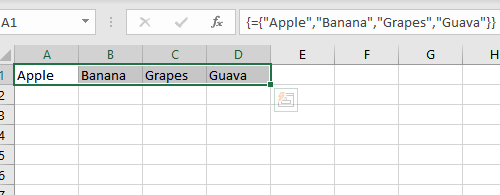The array created here is a horizontal array. You can also create a vertical array by changing the selected cells. You can create an array by specifying the starting and the ending positions, for example, if you wish to make an array of elements between A1 to F1 then you can write A1:F1. Now. let’s move to array formulas.

### Array formulas

Array formulas enable to process of several values and give several outputs. In a simple world, it can do multiple calculations and reduce a lot of human efforts. In other words, we can say that it solves array calculations and give an array as output too. Let’s understand more of them with an example, of students with their test marks,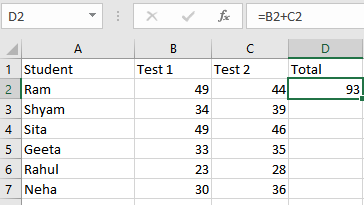Here, we have used a formula to evaluate the result in the ‘Total’ field. For inserting the formula, click on the cell where you want to store the answer and write the formula. Now, let’s try to calculate the same for all students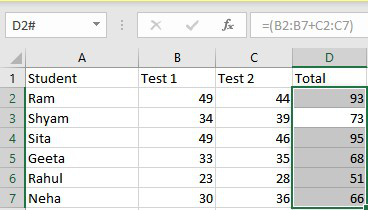For calculating the total for all the students, select the field where you want to store them, now select the range, and insert the formula. Array formulas are available in every version of Excel, so you don’t worry about your excel version. There are hundreds of operations possible using array formulas, which we will discuss in this article later. But before proceeding let’s understand what is the need to use an array formula.

Why use an Array Formula?

Array formulas are to most powerful yet most easy-to-use calculation tools which could be used to perform complex calculations and take place of hundreds of formulas with a single formula. You can further specify conditions for the calculation of data. Not just calculations you can also count the chars in cells, add date, day, or time, and even pick random data.

### How to insert an Array Formula?

Before entering the Array formula fir of all we understand some important points about the Array formula:

• After typing the formula you have to press keys CTRL+SHIFT+ENTER together. It will automatically change the normal formula into an array formula.
• If you manually type braces around the formula then it will not convert the formula into an array formula. You have to use CTRL+SHIFT+ENTER keys to convert the formula into an array formula.
• Whenever you edit the array formula the braces will disappear automatically and you have to again press the combination of CTRL+SHIFT+ENTER keys.
• If you forget to press the CTRL+SHIFT+ENTER keys then your formula will work as the normal formula.

Now let’s understand inserting an array formula.

Single Cell Array formula

Every array formula returns the result in either single or in multiple cells. So when an array formula returns its result in the single-cell then such type of formula is known as a single-cell array formula. Such types of formulas are SUM, AVERAGE, AGGREGATE, MAX, MIN, etc. Follow the following steps to use the single-cell array formula:

Step 1: Select the cell where you want to store the answer.

Step 2: Now go to the formula bar and write an “=” sign.

Step 3: Now to formulas (highlighted below), and select the operation you want to perform.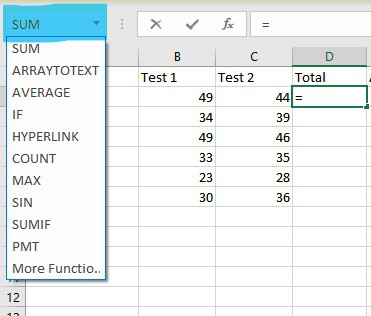Step 4: Here we want to perform the sum operation. Click on sum and a new popup will open. Write the cells you want to perform the operation with.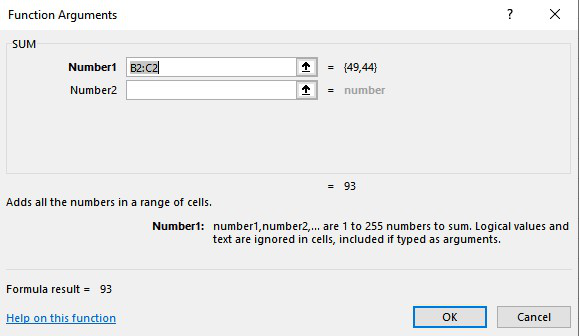#### Multi-Cell Array Formula

If you wish to perform the same calculation with more cells then you can use the multi-cell formula. You can implement it in two ways. Functions like TRANSPOSE, TREND, FREQUENCY, LINEST, etc are used for the multi-cell arrays. Implement the formula in single-cell by following the above steps. Now, drag the selection up to the cells you want to implement the formula. Below is the implementation of doing that.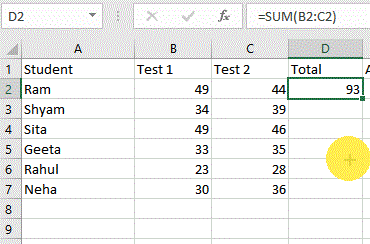Alternatively,

Specify the range of cells and an operator between them. For example, =(B1:B7 + C2:C7) and press Ctrl+Shift+Enter.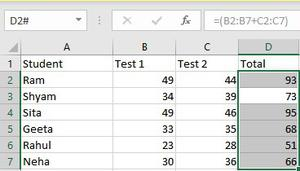### Excel Array Constants

Excel array contacts are a set of static values and can’t be changed. These values remain constant regardless of the operations performed on them. Now we will see how to create Array Constants in Excel and will perform different actions on them. There are three types of array constant available:

1. Horizontal or Row Array constant

A horizontal constant is present in a row. To create a horizontal constant we have to type multiple values separated by a comma in the enclosed braces. To enter a row array follow these steps below:

Step 1: Select the cells you want to work with.

Step 2: Go to the formula bar and start with an equal sign.

Step 3: Now enter the array you want to create in braces {}, separated by commas(,). Ex:- ={1,2,3,4,5}

Step 4: Now press Ctrl+Shift+Enter.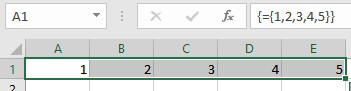2. Vertical or Column Array

Vertical array constants are generally present in Column Array. To create a vertical constant we have to type multiple values separated by a semicolon in the enclosed braces. To enter a column array follow these steps below:

Step 1: Select the cells you want to work with.

step 2: Go to the formula bar and start with an equal sign.

Step 3: Now enter the array you want to create in braces {} separated with termination sign(;). Ex:- ={“Coding”;”GFG”;”GeeksforGeeks”}

Step 4: Now press Ctrl+Shift+Enter.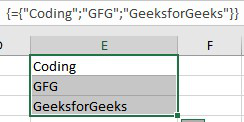3. 2-D array

In Excel, you are allowed to create a 2-D array. So to create a 2-D array constant, we have to type multiple values in which rows are separated by semicolons and d columns are separated by commas. Let’s understand the implementation of a 2-d array.

Step 1: Select the cells you want to work with.

Step 2: Go to the formula bar and start with an equal sign.

Step 3: Now enter the array you want to create in braces {} separated with commas(,) for entering the values in same row, termination sign(;) for row change. Ex:- ={1,2,3;”Coding”,”GFG”,”GeeksforGeeks”}

Step 4: Now press Ctrl+Shift+Enter.

### Working with Constant arrays

In the Excel formula, array constants are the important part. Some of the important concepts of Array constant are:

1. Elements: To create elements of array constant we have to follow the following points:

1. The elements of an array constant must be of numeric, alphabetic, boolean, or scientific notation and each of them is separated by commas or semicolons.
2. The elements of an array constant do not contain an array, cell reference, dates functions formulas, defined names, etc.
3. You can also be allowed to use text in the array of the element but the text is surrounded by hyphens(“”).

2. Naming Array Constants: You can name any array and use them as a variable for further references. For naming an array, follow the steps below:

1. Go to the formula tab. and select Define Name.
2. Write the array name in the name. You can also add some comments related to your array.
3. Click on OK.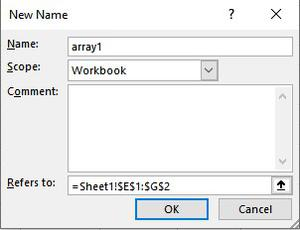3. Common Errors: Following are the common errors that will happen while working with array constants:

1. The use of delimiters in between the elements.
2. The selection of range is also an important point to remember.

### Unary Operator in Excel Array Formulas

Using unary operators we can process the array using AND and OR operators.

1. AND: It is denoted by an asterisk(*) symbol. It returns true if and only if all conditions are true,
2. OR: It is denoted by a plus(+) symbol. It returns true if any of the statement is true.

These operators are more useful when you have the same variable with a different value. For example, let’s consider a case, where data of 3 sellers of different months are given and you have to how much Shyam sold potatoes.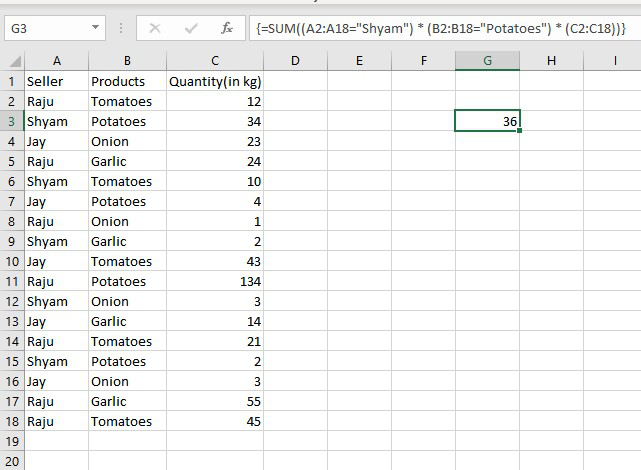My Personal Notes arrow_drop_up